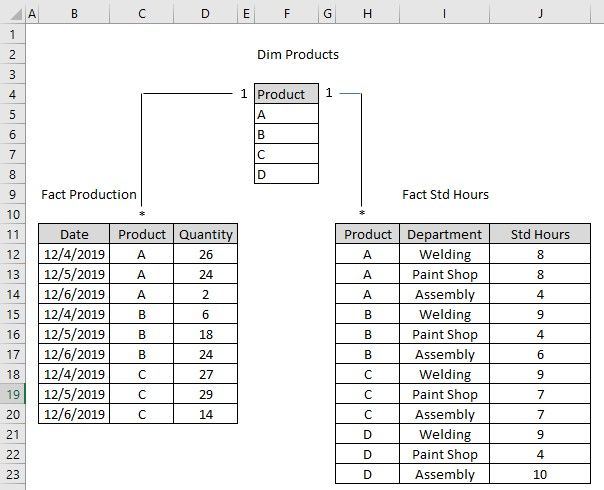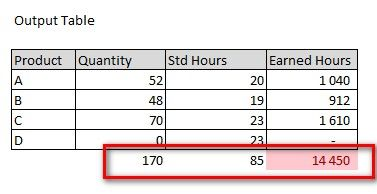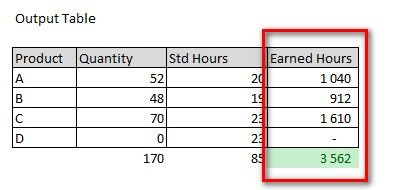cancel
Showing results for
Did you mean:Helper II

## Mulitply columns from 2 related tables

Hi, I'm trying to build a measure (called 'Earned Hours') that multiplies 2 columns from related tables - quantities and standard hours per product.

My data model looks like this:My current measure calculates correct results per row, but does not give me correct total:

Earned Hours = SUM('Fact Production'[Quantity])*SUM('Fact Std Hrs'[Std Hrs])The correct number should be:I have simplified my original post to make this easier to understand.

1 ACCEPTED SOLUTIONAnonymous
Not applicable

@Krzysztof_Hyla try below measure to get correct total

``````Measure =
VAR _product = SUM(factProduction[Quantity])*SUM(factStdHours[StdHours])
VAR _summarize = SUMMARIZE(dimProduct,dimProduct[Product],"total",SUM(factProduction[Quantity])*SUM(factStdHours[StdHours]))
RETURN IF(HASONEVALUE(dimProduct[Product]),_product,SUMX(_summarize,[total]))``````Anonymous
Not applicable

@Krzysztof_Hyla try below measure to get correct total

``````Measure =
VAR _product = SUM(factProduction[Quantity])*SUM(factStdHours[StdHours])
VAR _summarize = SUMMARIZE(dimProduct,dimProduct[Product],"total",SUM(factProduction[Quantity])*SUM(factStdHours[StdHours]))
RETURN IF(HASONEVALUE(dimProduct[Product]),_product,SUMX(_summarize,[total]))``````